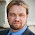Skip to main content

### A Stupid and Strange Way of Looking at Sports Power Ratings that could be Smart and Useful

As I've mentioned previously, a common method used in sports for estimating game outcomes known as log5 can be written $p = \frac{p_1 q_2}{p_1 q_2+q_1 p_2}$ where $$p_i$$ is the fraction of games won by team $$i$$ and $$q_i$$ is the fraction of games lost by team $$i$$. We're assuming that there are no ties. What's the easiest way to derive this estimate? Here's one argument. Assume team $$i$$ has a probability $$p_i$$ of beating an average team (a team that wins half its games). Now imagine that this means for any given game the team has some "strength" sampled from [0,1] with median $$p_i$$ and that the stronger team always wins. Thus, the probability that team 1 beats team 2 is $p = \int_0^1 \int_0^1 \! \mathrm{Pr}(p_1 > p_2) \, \mathrm{d} p_1 \mathrm{d} p_2 .$ This looks complicated, but but with probability $$p_1$$ team 1 is stronger than an average team and with probability $$p_2$$ team 2 is stronger than an average team. From this perspective the log5 estimate is just the Bayesian probability that team 1 will be stronger than an average team while team 2 will be weaker than an average team, conditional on either team 1 being stronger than an average team and team 2 weaker than an average team, or team 1 weaker than an average team and team 2 stronger than an average team. In these cases it's unambiguous which team is stronger. The cases where the strength of both teams is stronger or weaker than an average team (the ambiguous cases) are thus discarded.

How could this be useful? Instead of ignoring the ambiguous outcomes when estimating the outcome probabilities under this "latent strength" model, we could instead determine which probability distributions best fit the outcome distributions for a given league! Furthermore, this allows us to cohesively put a power rating system into a Bayesian framework by assigning to each team a Bayesian prior strength distribution. These priors could either be uninformative or informative using e.g. preseason rankings.

### Comments

1.This is interesting and seems to be closely related to the Bradley-Terry ranking model. I think the missing link in either of these approaches is connecting p_i with underlying cumulative strength of the team's individual players.

1.Yes, a Bayesian strength Bradley-Terry-Luce model would have $p= \frac{S_1}{S_1+S_2},$ where $$S_i$$ is sampled from some probability distribution. As I've mentioned in a previous article, these approaches may be related by setting $$S_i = \frac{p_i}{q_i}$$.

### A Bayes' Solution to Monty Hall

For any problem involving conditional probabilities one of your greatest allies is Bayes' Theorem. Bayes' Theorem says that for two events A and B, the probability of A given B is related to the probability of B given A in a specific way.

Standard notation:

probability of A given B is written $$\Pr(A \mid B)$$
probability of B is written $$\Pr(B)$$

Bayes' Theorem:

Using the notation above, Bayes' Theorem can be written: $\Pr(A \mid B) = \frac{\Pr(B \mid A)\times \Pr(A)}{\Pr(B)}$Let's apply Bayes' Theorem to the Monty Hall problem. If you recall, we're told that behind three doors there are two goats and one car, all randomly placed. We initially choose a door, and then Monty, who knows what's behind the doors, always shows us a goat behind one of the remaining doors. He can always do this as there are two goats; if we chose the car initially, Monty picks one of the two doors with a goat behind it at random.

Assume we pick Door 1 and then Monty sho…

### Mixed Models in R - Bigger, Faster, Stronger

When you start doing more advanced sports analytics you'll eventually starting working with what are known as hierarchical, nested or mixed effects models. These are models that contain both fixed and random effects. There are multiple ways of defining fixed vs random random effects, but one way I find particularly useful is that random effects are being "predicted" rather than "estimated", and this in turn involves some "shrinkage" towards the mean.

Here's some R code for NCAA ice hockey power rankings using a nested Poisson model (which can be found in my hockey GitHub repository):
model <- gs ~ year+field+d_div+o_div+game_length+(1|offense)+(1|defense)+(1|game_id) fit <- glmer(model, data=g, verbose=TRUE, family=poisson(link=log) ) The fixed effects are year, field (home/away/neutral), d_div (NCAA division of the defense), o_div (NCAA division of the offense) and game_length (number of overtime periods); off…

### Notes on Setting up a Titan V under Ubuntu 17.04

I recently purchased a Titan V GPU to use for machine and deep learning, and in the process of installing the latest Nvidia driver's hosed my Ubuntu 16.04 install. I was overdue for a fresh install of Linux, anyway, so I decided to upgrade some of my drives at the same time. Here are some of my notes for the process I went through to get the Titan V working perfectly with TensorFlow 1.5 under Ubuntu 17.04.

Old install:
Ubuntu 16.04
EVGA GeForce GTX Titan SuperClocked 6GB
2TB Seagate NAS HDD
+ additional drives

New install:
Ubuntu 17.04
Titan V 12GB
/ partition on a 250GB Samsung 840 Pro SSD (had an extra around)
/home partition on a new 1TB Crucial MX500 SSD
New WD Blue 4TB HDD
+ additional drives

You'll need to install Linux in legacy mode, not UEFI, in order to use Nvidia's proprietary drivers for the Titan V. Note that Linux will cheerfully boot in UEFI mode, but will not load any proprietary drivers (including Nvidia's). You'll need proprietary drivers for Tens…